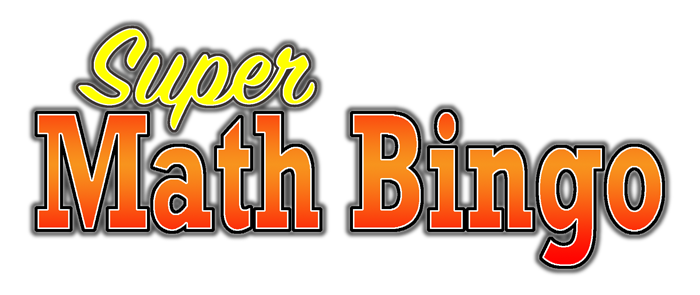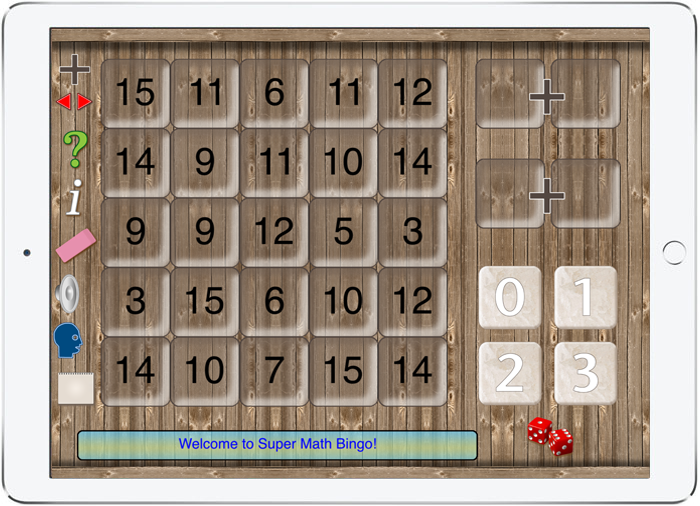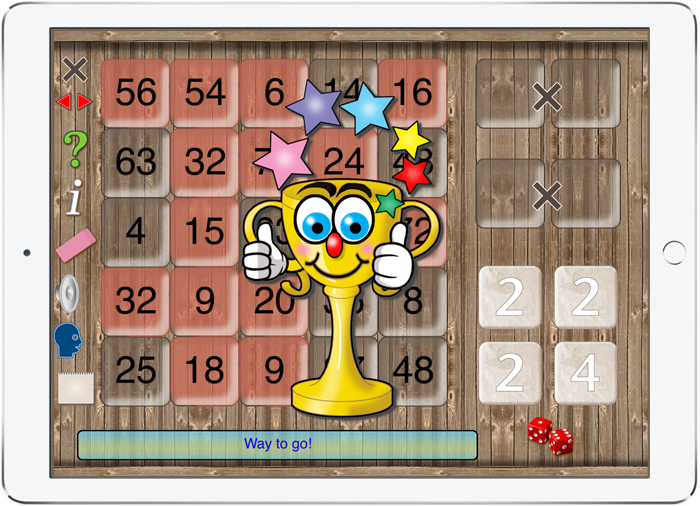Practice Basic Arithmetic Skills with Super Math Bingo...An Interactive Math Activity to Help Improve Basic Math Skills...The bingo board shown above is used to help students practice arithmetic skills. Tiles are moved in order to make mathematical expressions. The goal is to completely mark a column or row on the board. The player earns a trophy when reaching the goal. Super Math Bingo is a variation on the popular bingo game but it has a mathematical twist, the object is to make addition, subtraction or multiplication expressions in order to achieve a bingo. Super Math Bingo app introduces students to a simple game that encourages them to practice their mental math skills. The gameboard consists of a 5x5 grid. There are three ways to play the game: 1. AdditionMode where the object is to make sums by adding two numbers between 0 and 9. 2. Subtraction Mode where the object is to find the difference between two numbers up to 20. 3. Multiplication Mode where the object is to make products up to 9x9. The goal is to completely mark either a row or column on the bingo board. This app challenges the player to think about arithmetic combinations and to develop a strategy for making a bingo. Playing the game involves sliding number tiles to make mathematical expressions. Special sound effects and synthesized speech make the game fun to use. The interactive design helps student practice basic arithmetic operations and also facilitates the development of logical thinking strategies. [ See also Geometry Portal AR, Algebra Portal AR and Geometry AR ]Special features make using the Super Math Bingo app fun: 1. Slide numbers into place to develop a winning strategy. 2. Practice Basic Arithmetic Skills using Addition, Subtraction, and Multiplication. 3. Speech and sound effects enhance the game. 4. Available for iPad iOS 11.\$0.99 Now Available on the Apple App Store.